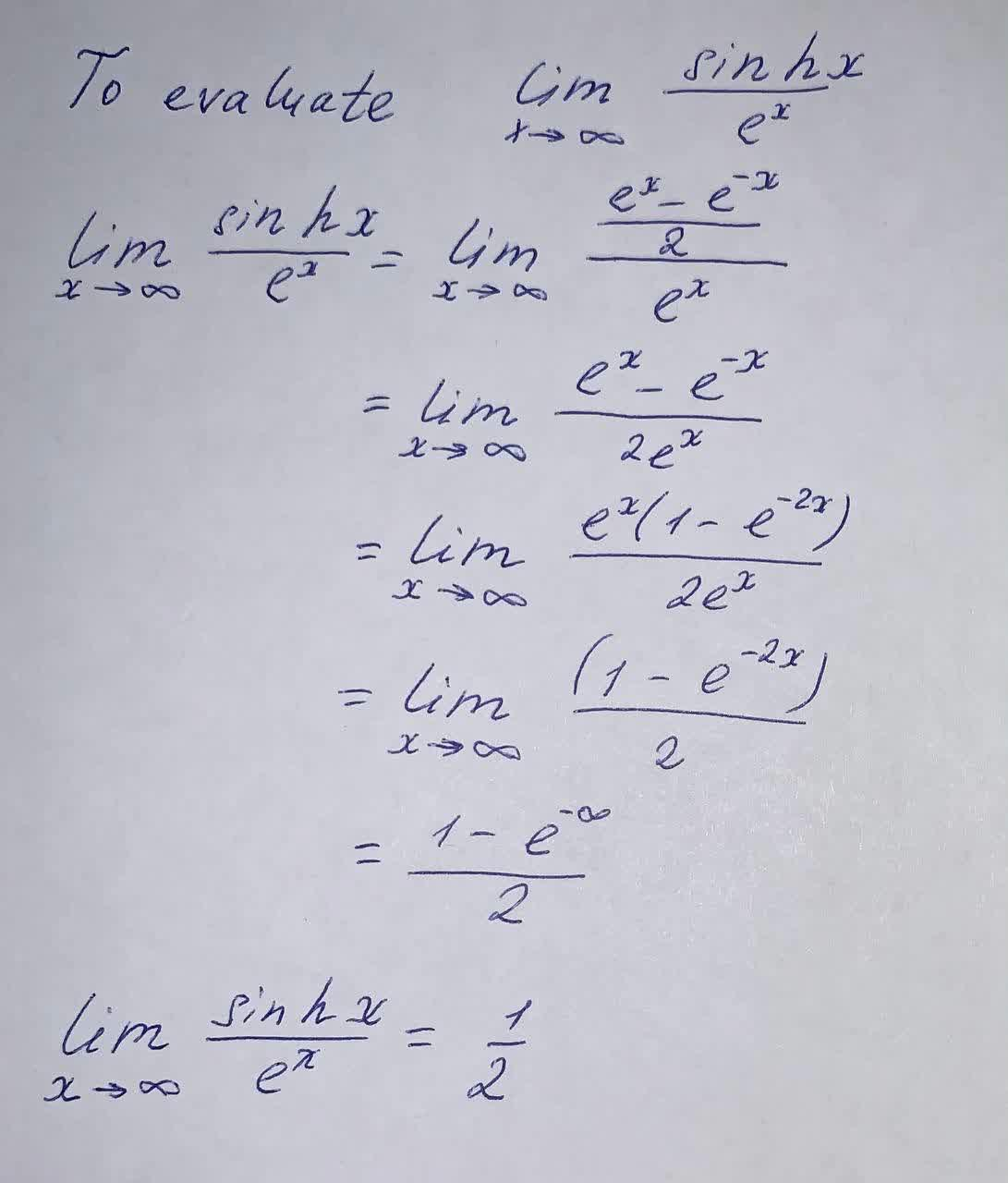Question# Evaluate \lim_{x \rightarrow \infty} \frac{\sin h x}{e^x}

Limits and continuity
ANSWEREDEvaluate $$\displaystyle\lim_{{{x}\rightarrow\infty}}{\frac{{{\sin{{h}}}{x}}}{{{e}^{{x}}}}}$$